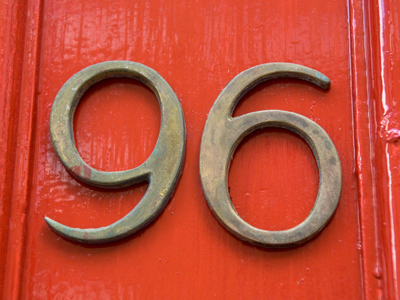12 x 8 = 96.

# 8 Times Table

Learning the times tables is a vital tool when multiplying numbers. By the end of Year 2 children are often fluent in their times tables up to 10. When they enter into KS2 in Year 3 they will also be taught some higher times tables in their Maths and Numeracy lessons. By the end of KS2 they will be familiar with all of the times tables from one to twelve. This quiz will test their knowledge of the 8 times table.

Learning the 8 times table is all about multiplying numbers by 8. The larger the numbers, the harder it becomes. Learning the 8 times table until you know the answer before you even think about it will really help you when going shopping and multiplying numbers in everyday life.

Play this quiz to test your knowledge of the 8 times table.

1.
6 x 8 =
44
48
52
60
2.
9 x 8 =
70
72
74
78
To multiply a number by 9 first times it by 10 and then take it away, so 8 x 10 = 80 and 80 - 8 = 72
3.
11 x 8 =
18
19
80
88
10 x 8 = 80 and 80 + 8 = 88
4.
2 x 8 =
10
12
16
20
5.
12 x 8 =
96
108
116
128
6.
3 x 8 =
22
24
27
29
There are 24 hours in a day - that's 3 lots of 8 hours
7.
8 x 8 =
56
60
64
66
8.
4 x 8 =
18
28
32
37
A fully grown adult has 32 teeth - so long as they haven't lost any!
9.
5 x 8 =
13
18
20
40
To times a number by 5, first times it by 10 and then half it, so 8 x 10 = 80 and 80 ÷ 2 = 40
10.
7 x 8 =
56
58
62
64